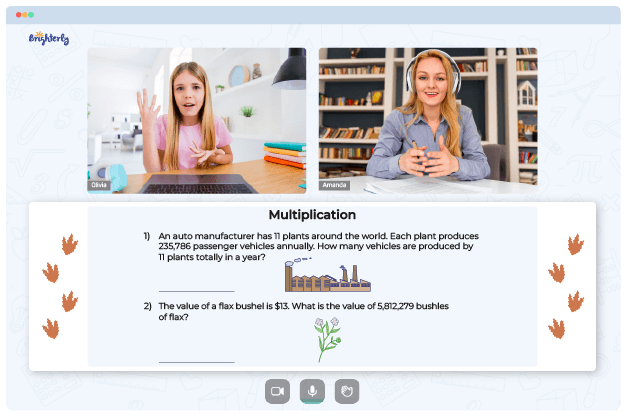# Multiplying Fractions By Whole Numbers Worksheets

Multiplying fractions by whole numbers is a complex process involving different math concepts. It can be confusing for kids, so when teaching a child, you must introduce worksheets as a tool to help them practice.

Multiplying fractions by whole numbers worksheets will help better understand the relationship between fractions and multiplications. These worksheets are also fun to study with, making kids more interested in solving math problems and replicating that zeal in the classroom.

## Benefits of using multiplication of fractions and whole numbers worksheets

There are benefits of multiplying fractions with whole numbers worksheets, and here are some of them.

### Worksheets simplify multiplication

When you first attempt multiplying a fraction by a whole number, you realize that solving such problem is not an easy process. Even when setting classwork, you might need to explain the process repeatedly; at some point, it may become too much. So, you can imagine what students think of it too.

You can’t simplify the process in one lesson, so you need a worksheet. With suitable tolls, kids can go over the problem independently and learn to break down the process.

### They encourage analytical thinking

When a child uses a multiply fractions by whole numbers worksheet, they experience firsthand how to take apart a problem and analyze it. To multiply fractions by whole numbers, you must use different strategies to solve separate elements of the problem and come to a final answer. Consistent use of worksheets can ensure that students learn how to deal with math problems analytically, notwithstanding the question.

Math for Kids

Is Your Child Struggling With Math?
1:1 Online Math Tutoring### The worksheets teach the kids to use fractions

Fractions are used in many math problems, including algebraic equations, ratios, proportions, probability, etc. And unless they appear as standalone questions, kids won’t know how to apply fractions in problems that do not have them at the beginning. With the multiplying fractions with whole numbers worksheets, kids can learn how to apply fractions to all the other math concepts required.

### They are easy to use

Unlike class works and homework set randomly, multiplying fractions by whole numbers worksheets are easy for kids to adopt. They include instructions that students can follow to solve the problems.

Some worksheets even come with answers attached, including a breakdown of how the educators got to the answer. Kids love the tools, which makes your job as a math teacher or concerned parent easy.### Multiplying Fractions By Whole Numbers Worksheets PDF

Multiply Fractions By Whole Numbers Worksheet### Multiplying Fractions By Whole Numbers Worksheets PDF

Multiplying Fractions With Whole Numbers Worksheets### Multiplying Fractions By Whole Numbers Worksheets PDF

Multiplying Fractions By Whole Numbers Worksheets 5Th Grade### Multiplying Fractions By Whole Numbers Worksheets PDF

Multiplying Fractions And Whole Numbers Worksheet

## Multiplying Fractions by Whole Numbers Worksheets in PDF

If you like what you’ve read, you can search math websites to get 5th grade multiplying fractions with whole numbers worksheets for your kids. There are so many kinds of worksheets online, and they are printable.

The best part is that the worksheets are free to download. So, try as many as possible, especially the colorful ones that will grab your students’ attention and keep them focused on solving the problems in the worksheets.

### Fractions Worksheets

Need help with Multiplication?• Does your child struggle with understanding the concept of multiplication?
• Try learning with an online tutor.

Is your child having trouble mastering multiplication? An online tutor could provide the necessary assistance.